# True Range and How It Differs from Range

The concept of true range and calculation of ATR (Average True Range) is confusing for many people, as you are actually comparing three values instead of applying one exact formula. This page is a detailed guide to calculation of true range. I've tried to attach a few simple chart examples to better illustrate the point.

## How True Range Is Different from Range

The classical range (of a trading day or price chart bar) is the difference between that bar's high and low: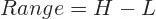It is fast and easy, but it has one drawback: It only measures intraday/intrabar volatility and fails to look at the volatility between individual bars.

Sometimes there are gaps, when the market opens much higher or much lower than the previous close and doesn't go back to it at any point throughout the session. In other words, the previous day's close is outside the current day's trading range. While classical range (high minus low) can't measure these kinds of gaps, true range can.

## True Range Formula

True range is the maximum of three price ranges: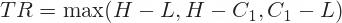... where:

• H = high of current bar
• L = low of current bar
• C1 = close of previous bar

## 1) Typical Situations when True Range = High less Low

Very often true range equals the traditional range. This is when the distance between high and low (the traditional range) is greater than both

• the distance of high H from previous bar's close C1 and
• the distance of Low L from previous bar's close.

This happens when the close of the previous bar is within the current bar's range. See the picture below.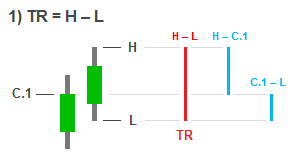## 2) Typical Situations when True Range = High less Previous Close

The second type of situation is when the previous bar's close C1 is lower than current bar's low L. When this happens, the distance between current bar's high and previous bar's close is greater than the distance between current bar's high and low. Therefore true range equals high less previous close. This is the case any time when the new bar opens above the previous bar's close and the price doesn't get below it during the whole bar duration.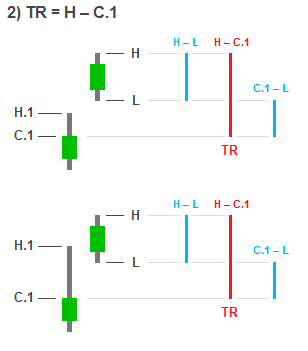## Previous Bar's High or Low Are Not Important

Note that the previous bar's high H1 is not important at all for true range. In the picture above you see two different situations when true range equals high less previous close. It doesn't matter whether the high of the first bar H1 is lower or higher than current bar's low L. In the situation on the picture, the high of the first bar could have been even higher than the second bar's high, but true range at the second bar would still be the same. Neither high nor low of the previous bar enter the true range calculations in any way.

True range only measures what happens from the moment of previous close until the current bar close. Previous bar high and low do not fall inside that period.

## 3) Typical Situations when True Range = Previous Close less Low

Finally, let's look at the third possible situation. When previous bar's close C1 is higher than current bar's high H, true range equals previous bar's close less current bar's low L. An example of such situation would be a "gap down" trading day. Again, the low of the first bar L1 is unimportant for the second bar's true range.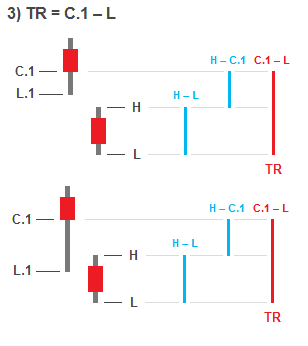## True Range in Another (Maybe Simpler) Way

There are only three numbers you need to know for determining true range:

1. Previous bar's close C1
2. Current bar's high H
3. Current bar's low L

True Range is the greatest distance you can find between any two of these three prices. In other words, it is the greatest of the absolute values of the three differences between the three prices, or the greatest of:

• High minus low H – L
• High minus previous close H – C1
• Previous close minus low C1 – L

## Calculating Average True Range

Most frequently the concept of true range is used in the smoothed form of Average True Range (ATR), which is an indicator calculated as moving average of true range over a number of days or periods (see how to calculate true range and ATR in Excel). The period length used in this calculation is most commonly 14 or 20, but it can be any number greater than 1.

True range and Average True Range were first described by J. Welles Wilder Jr. in his 1978 book New Concepts in Technical Trading Systems.

ATR is widely used to assess volatility conditions in the market. Typical applications of ATR include risk management, determining position size, or setting stop-loss order distance as a multiple of ATR.

By remaining on this website or using its content, you confirm that you have read and agree with the Terms of Use Agreement.

We are not liable for any damages resulting from using this website. Any information may be inaccurate or incomplete. See full Limitation of Liability.

Content may include affiliate links, which means we may earn commission if you buy on the linked website. See full Affiliate and Referral Disclosure.

We use cookies and similar technology to improve user experience and analyze traffic. See full Cookie Policy.